This is “Linear Inequalities (One Variable)”, section 2.8 from the book Beginning Algebra (v. 1.0). For details on it (including licensing), click here.

Has this book helped you? Consider passing it on:
Creative Commons supports free culture from music to education. Their licenses helped make this book available to you.
DonorsChoose.org helps people like you help teachers fund their classroom projects, from art supplies to books to calculators.

## 2.8 Linear Inequalities (One Variable)

### Learning Objectives

1. Identify linear inequalities and check solutions.
2. Solve linear inequalities and express the solutions graphically on a number line and in interval notation.
3. Solve compound linear inequalities and express the solutions graphically on a number line and in interval notation.
4. Solve applications involving linear inequalities and interpret the results.

## Definition of a Linear Inequality

A linear inequalityA mathematical statement relating a linear expression as either less than or greater than another. is a mathematical statement that relates a linear expression as either less than or greater than another. The following are some examples of linear inequalities, all of which are solved in this section: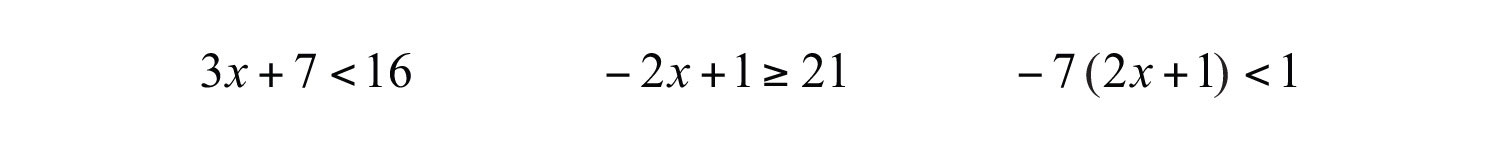A solution to a linear inequalityA real number that produces a true statement when its value is substituted for the variable. is a real number that will produce a true statement when substituted for the variable. Linear inequalities have either infinitely many solutions or no solution. If there are infinitely many solutions, graph the solution set on a number line and/or express the solution using interval notation.

Example 1: Are $x=−2$ and $x=4$ solutions to $3x+7<16$?

Solution: Substitute the values for x, simplify, and check to see if we obtain a true statement.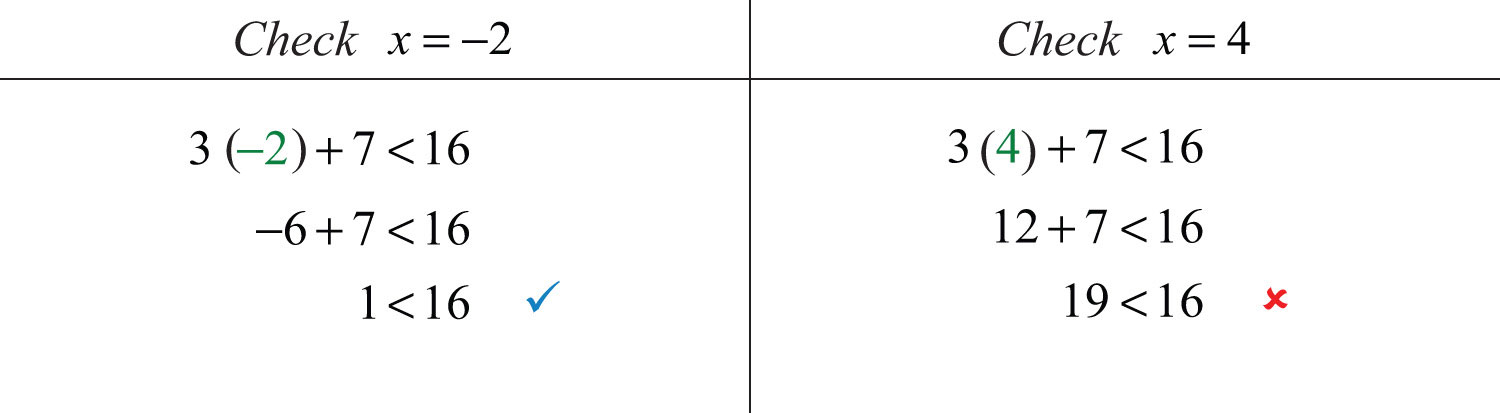Answer: $x=−2$ is a solution and $x=4$ is not.

## Algebra of Linear Inequalities

All but one of the techniques learned for solving linear equations apply to solving linear inequalities. You may add or subtract any real number to both sides of an inequality, and you may multiply or divide both sides by any positive real number to create equivalent inequalities. For example,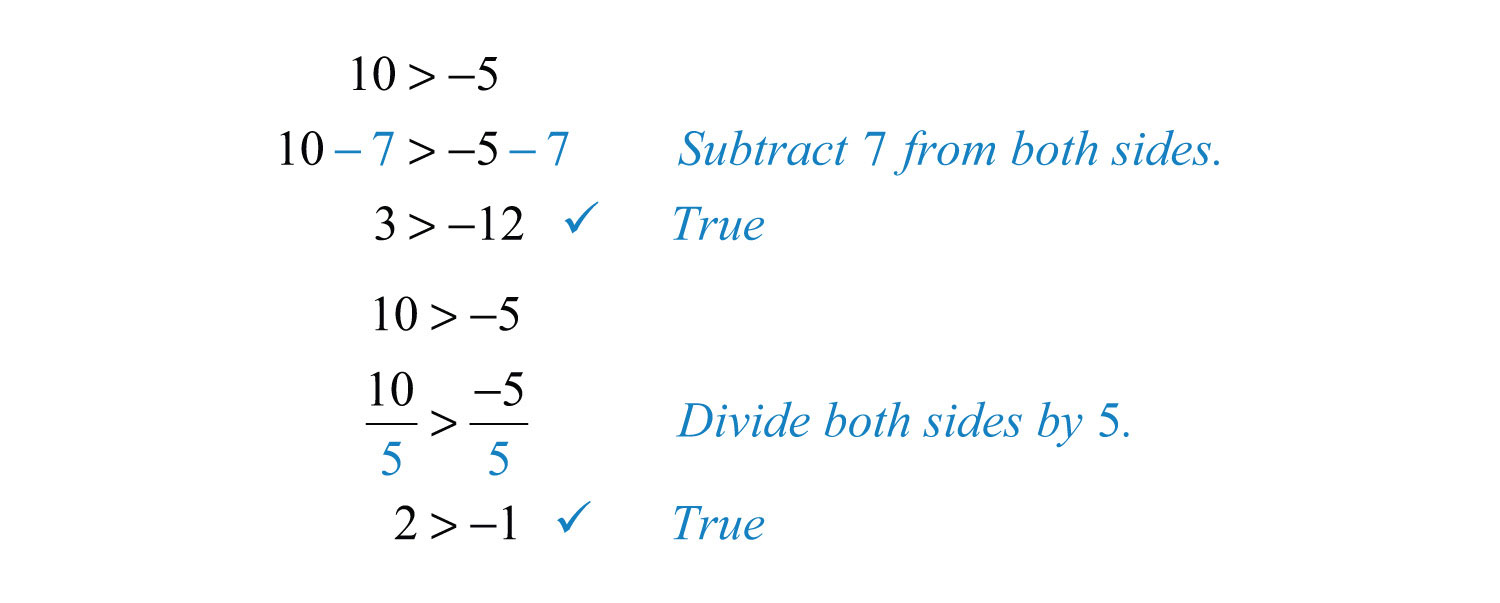Both subtracting 7 from each side and dividing each side by +5 results in an equivalent inequality that is true.

Example 2: Solve and graph the solution set: $3x+7<16$.

Solution: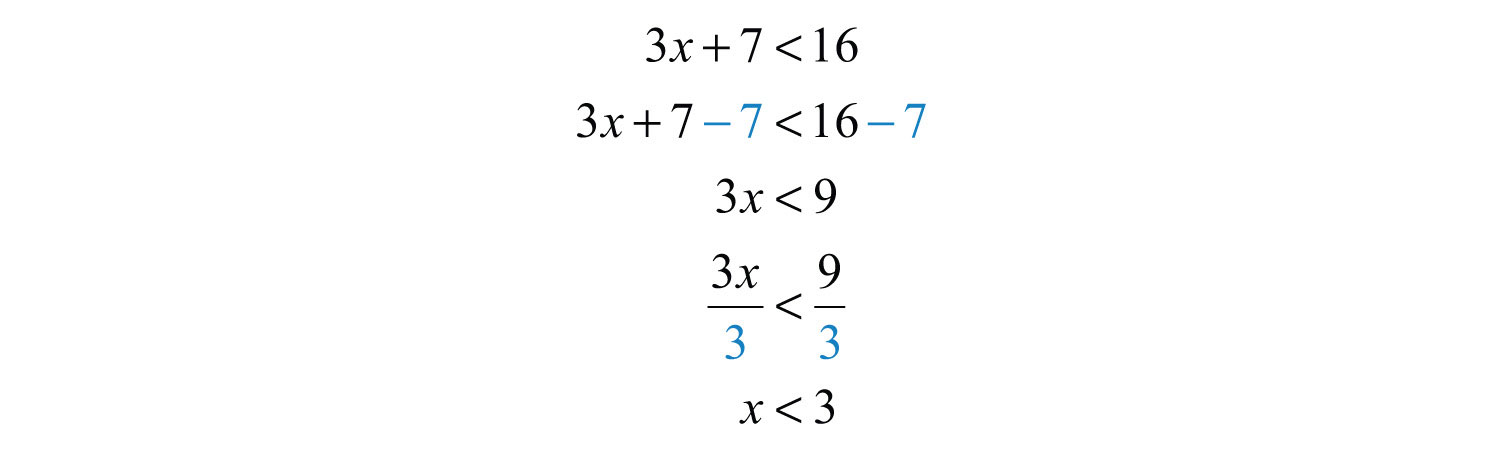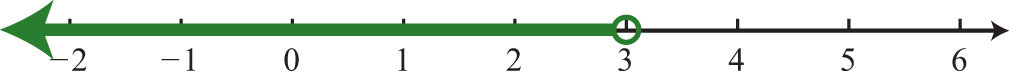It is helpful to take a minute and choose a few values in and out of the solution set, substitute them into the original inequality, and then verify the results. As indicated, you should expect $x=0$ to solve the original inequality, but $x=5$ should not.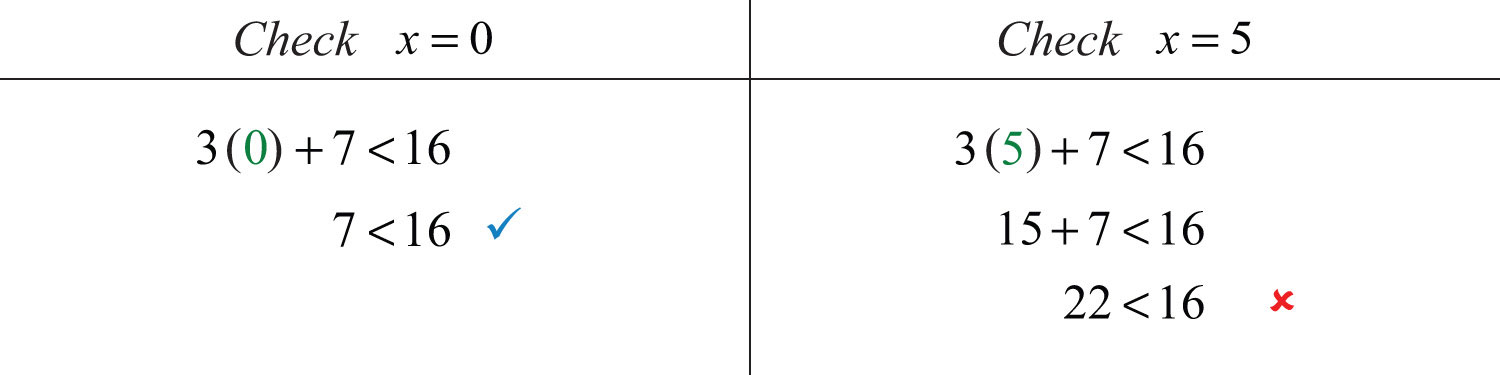Checking in this manner gives a good indication that the inequality is solved correctly. This can be done mentally.

Answer: Interval notation: $(−∞, 3)$

When working with linear inequalities, a different rule applies when multiplying or dividing by a negative number. To illustrate the problem, consider the true statement $10>−5$ and divide both sides by −5.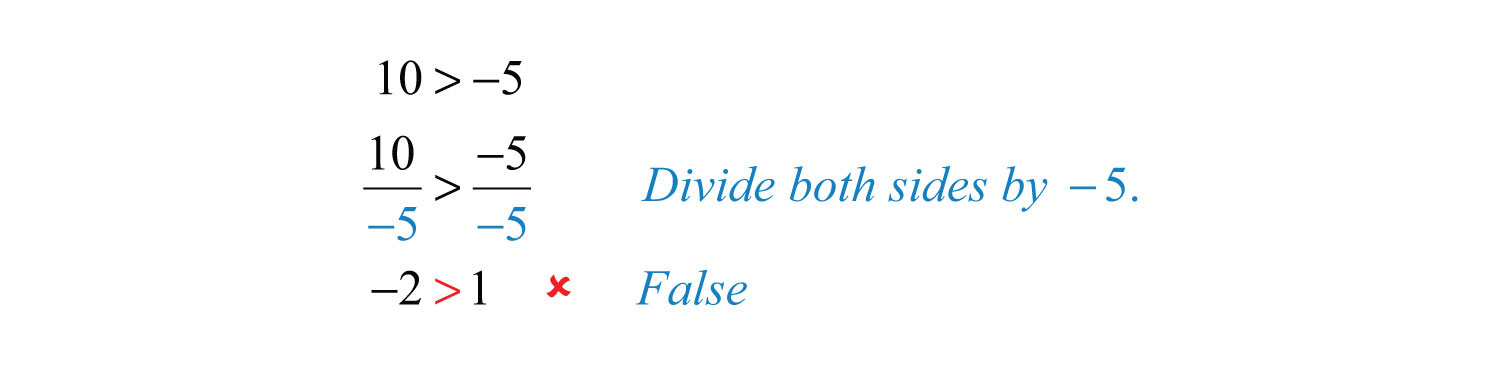Dividing by −5 results in a false statement. To retain a true statement, the inequality must be reversed.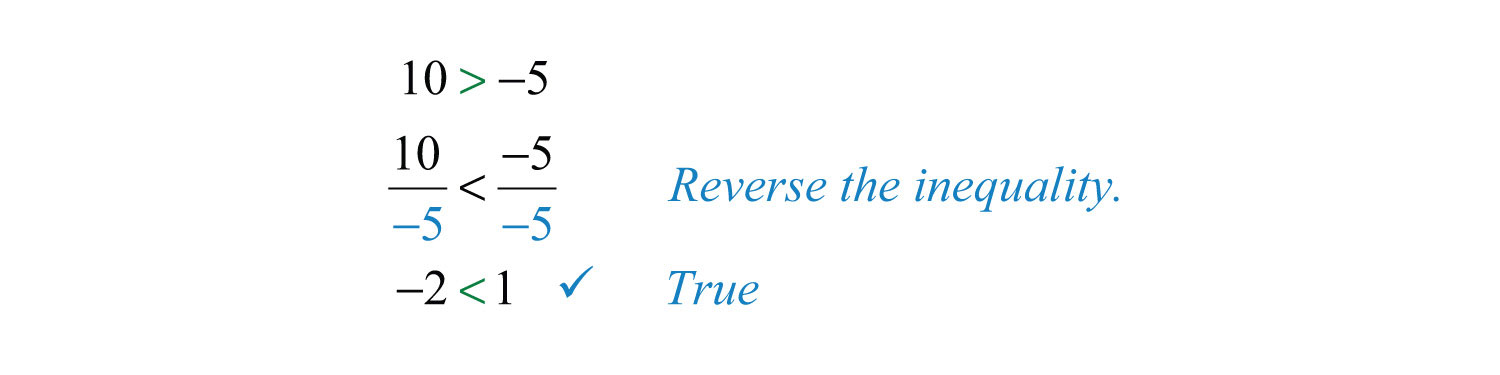The same problem occurs when multiplying by a negative number. This leads to the following new rule: when multiplying or dividing by a negative number, reverse the inequality. It is easy to forget to do this so take special care to watch for negative coefficients.

In general, given algebraic expressions A and B, where c is a positive nonzero real number, we have the following properties of inequalitiesProperties used to obtain equivalent inequalities and used as a means to solve them.: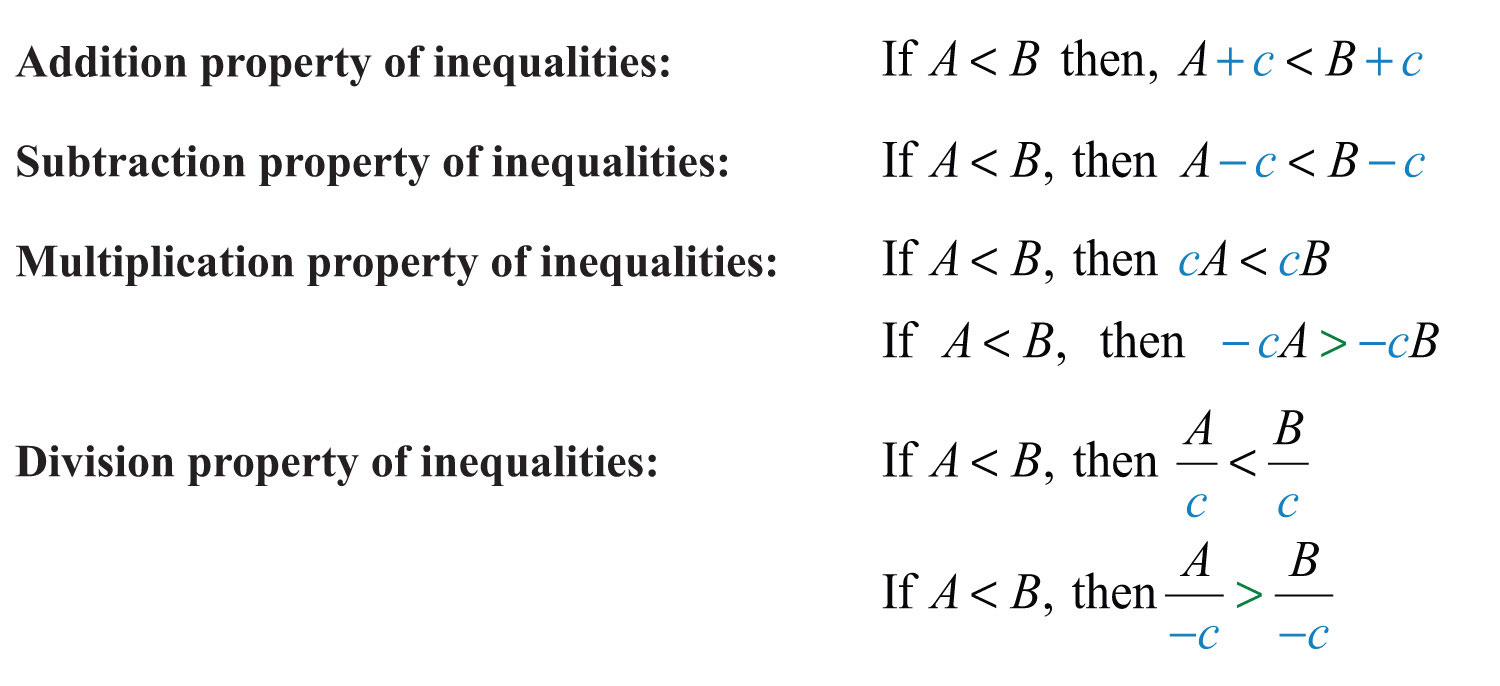We use these properties to obtain an equivalent inequalityInequalities that share the same solution set., one with the same solution set, where the variable is isolated. The process is similar to solving linear equations.

Example 3: Solve: $−2x+1≥21$.

Solution: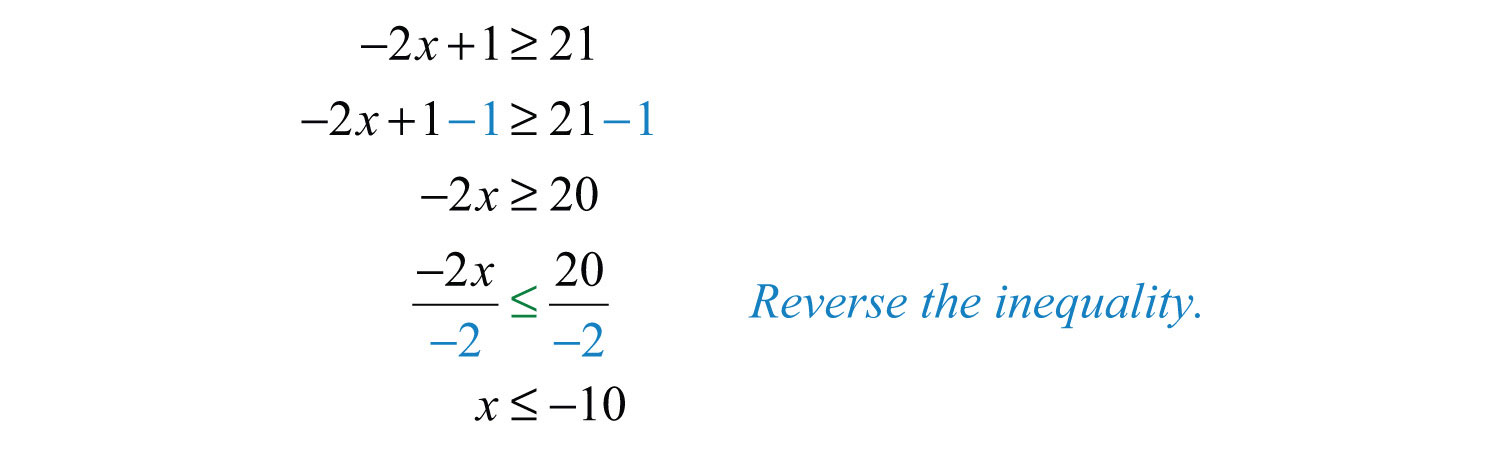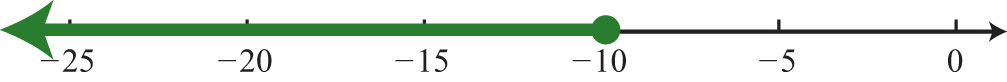Answer: Interval notation: $(−∞, −10]$

Example 4: Solve: $−7(2x+1)<1$.

Solution: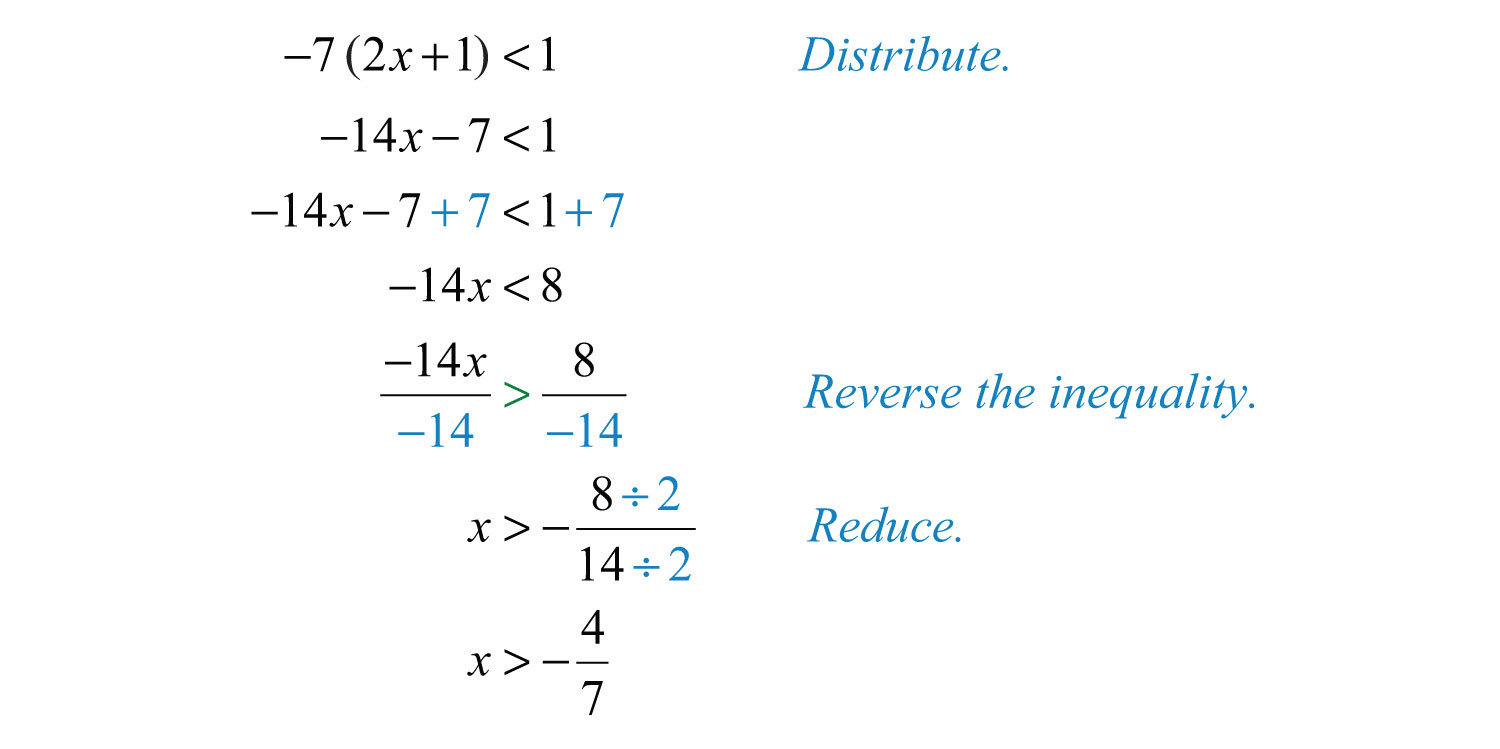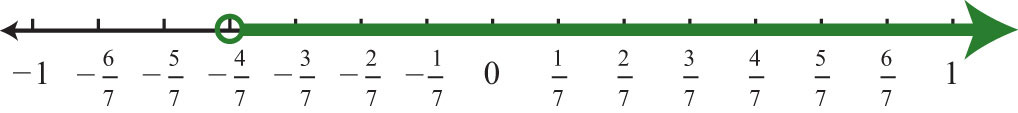Answer: Interval notation: $(−47, ∞)$

Example 5: Solve: $5x−3(2x−1)≥2(x−3)$.

Solution: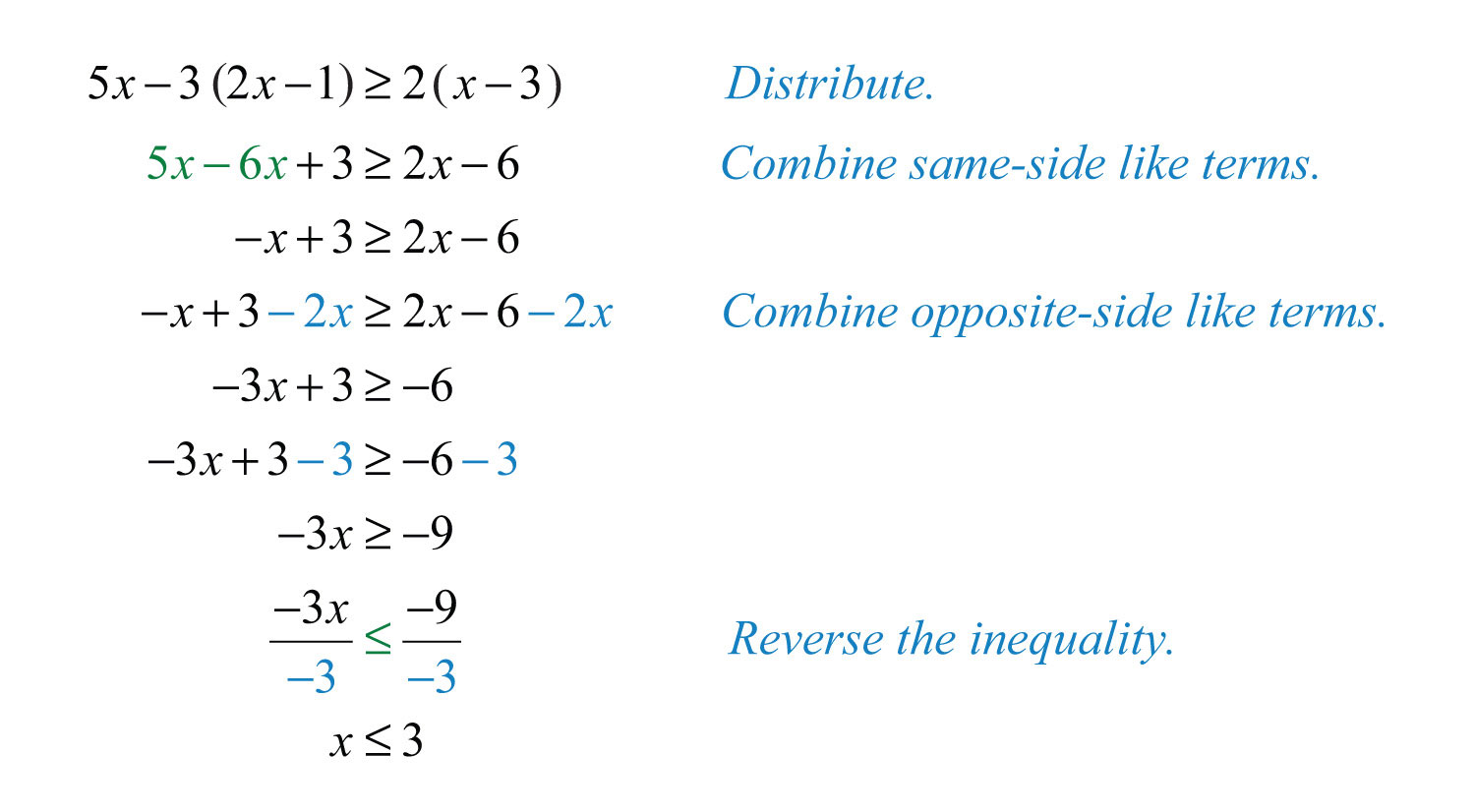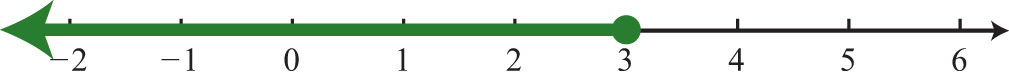Answer: Interval notation: $(−∞, 3]$

Try this! Solve: $3−5(x−1)≤28$.

Answer: $[−4, ∞)$

### Video Solution

(click to see video)

## Compound Inequalities

Following are some examples of compound linear inequalities: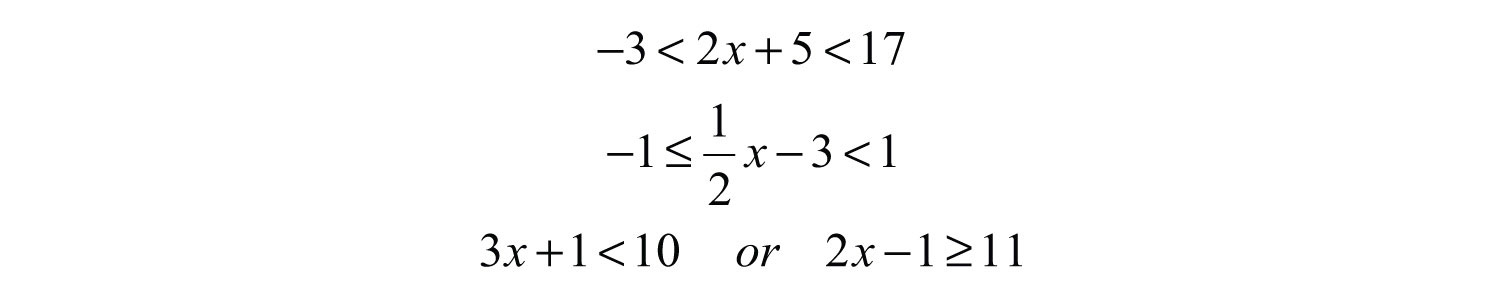These compound inequalitiesTwo or more inequalities in one statement joined by the word “and” or by the word “or.” are actually two inequalities in one statement joined by the word “and” or by the word “or.” For example,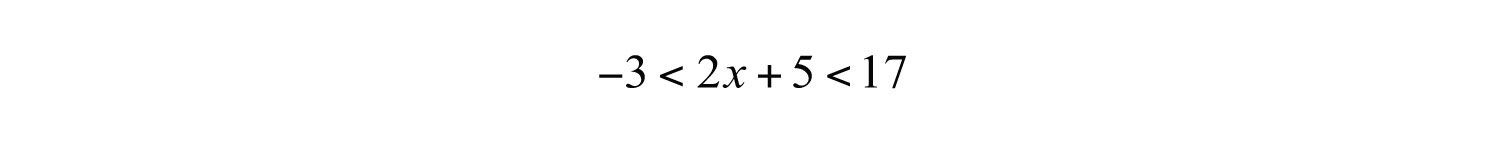is a compound inequality because it can be decomposed as follows: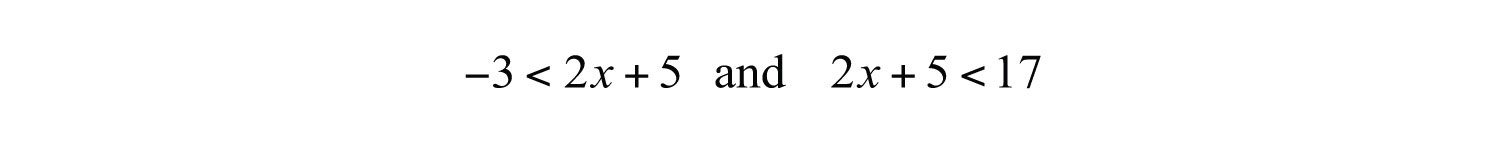Solve each inequality individually, and the intersection of the two solution sets solves the original compound inequality. While this method works, there is another method that usually requires fewer steps. Apply the properties of this section to all three parts of the compound inequality with the goal of isolating the variable in the middle of the statement to determine the bounds of the solution set.

Example 6: Solve: $−3<2x+5<17$.

Solution: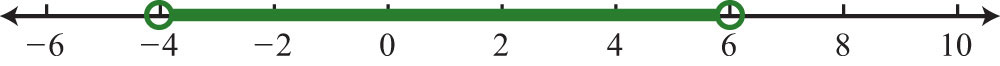Example 7: Solve: $−1≤12x−3<1$.

Solution: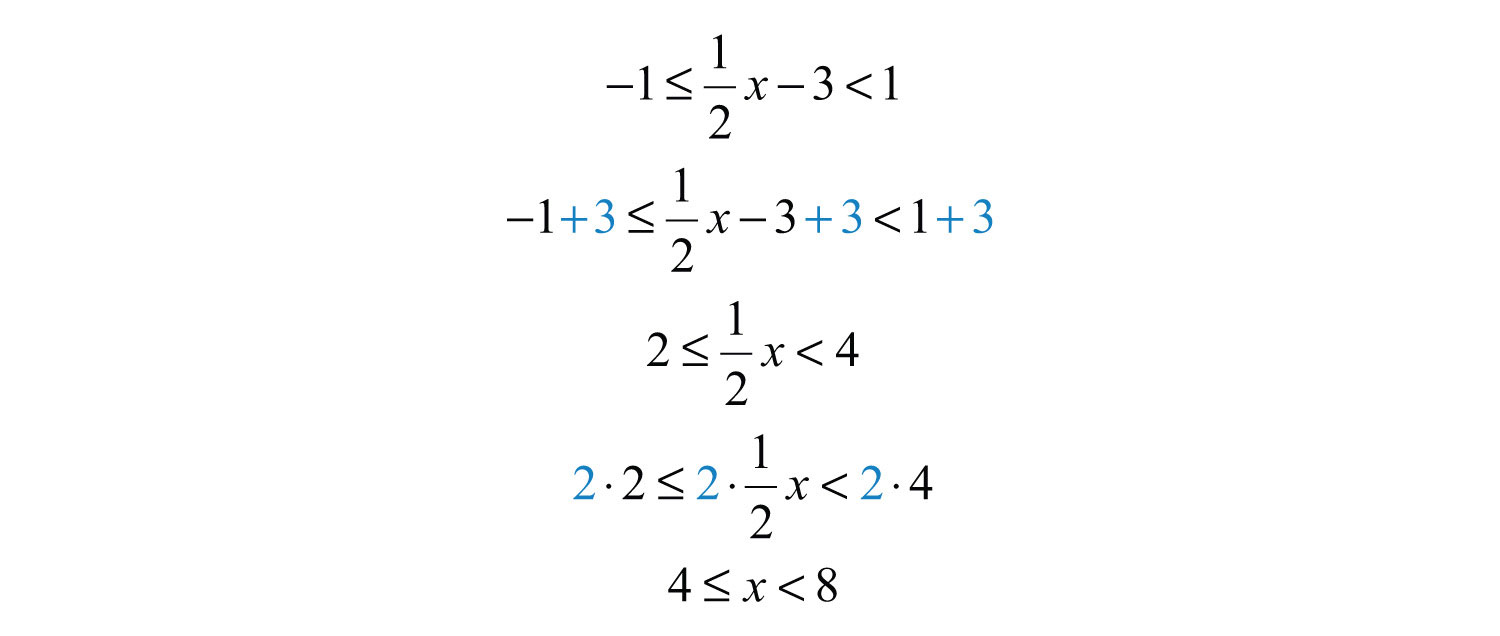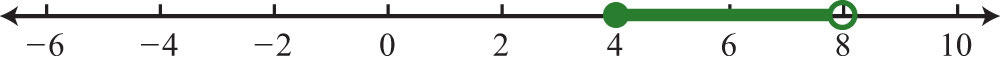It is important to note that when multiplying or dividing all three parts of a compound inequality by a negative number, you must reverse all of the inequalities in the statement. For example,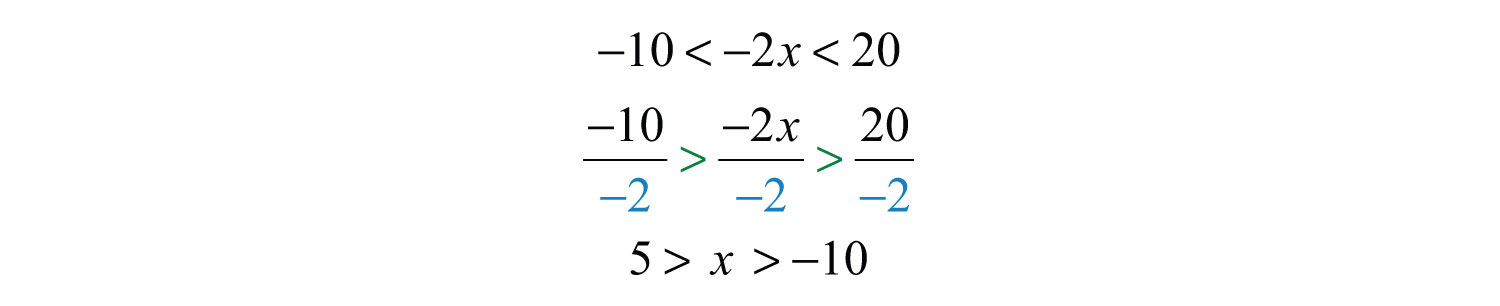The answer above can be written in an equivalent form, where smaller numbers lie to the left and the larger numbers lie to the right, as they appear on a number line.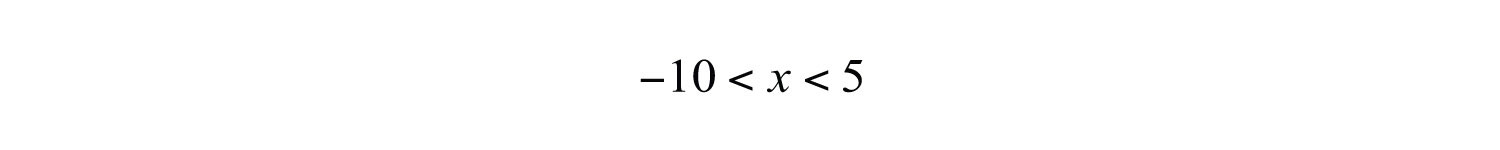Using interval notation, write $(−10, 5)$.

Try this! Solve: $−8≤2(−3x+5)<34$.

Answer: $(−4, 3]$

### Video Solution

(click to see video)

For compound inequalities with the word “or” you must work both inequalities separately and then consider the union of the solution sets. Values in this union solve either inequality.

Example 8: Solve:

Solution: Solve each inequality and form the union by combining the solution sets.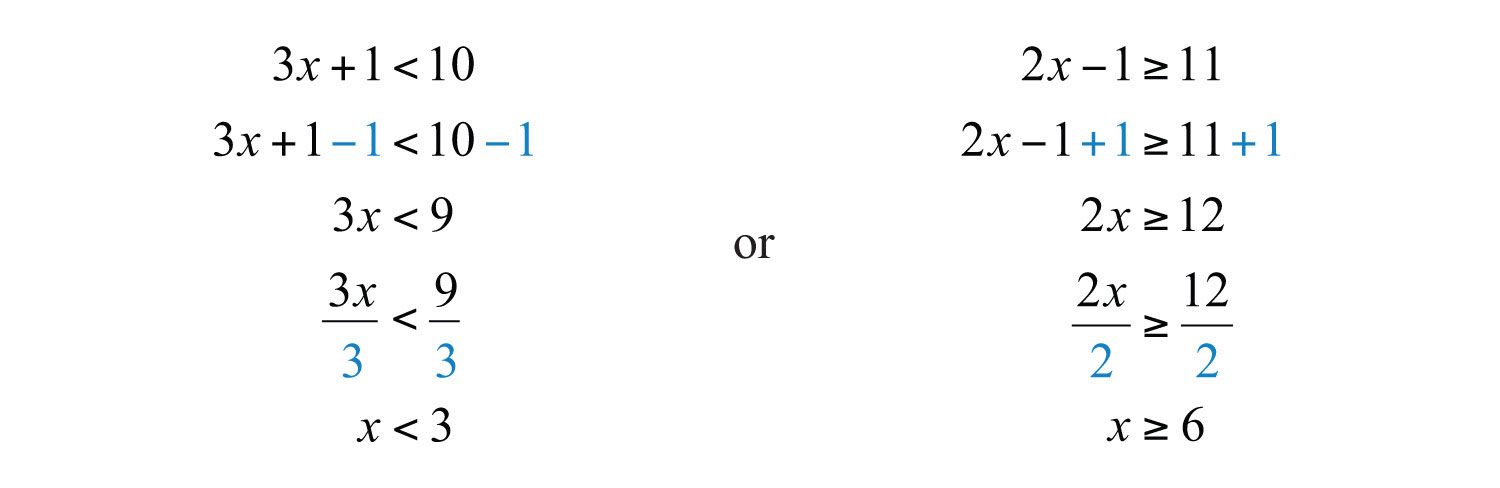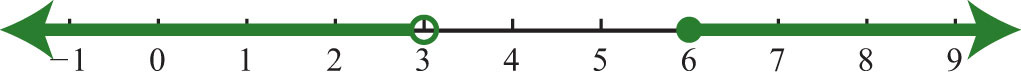Answer: Interval notation: $(−∞, 3)∪[6, ∞)$

Try this! Solve: .

Answer: $(−∞,−1)∪(32, ∞)$

### Video Solution

(click to see video)

## Applications of Linear Inequalities

Some of the key words and phrases that indicate inequalities are summarized below:

Key Phrases Translation
A number is at least 5. $x≥5$
A number is 5 or more inclusive.
A number is at most 3. $x≤3$
A number is 3 or less inclusive.
A number is strictly less than 4. $x<4$
A number is less than 4, noninclusive.
A number is greater than 7. $x>7$
A number is more than 7, noninclusive.
A number is in between 2 and 10. $2
A number is at least 5 and at most 15. $5≤x≤15$
A number may range from 5 to 15.

As with all applications, carefully read the problem several times and look for key words and phrases. Identify the unknowns and assign variables. Next, translate the wording into a mathematical inequality. Finally, use the properties you have learned to solve the inequality and express the solution graphically or in interval notation.

Example 9: Translate: Five less than twice a number is at most 25.

Solution: First, choose a variable for the unknown number and identify the key words and phrases.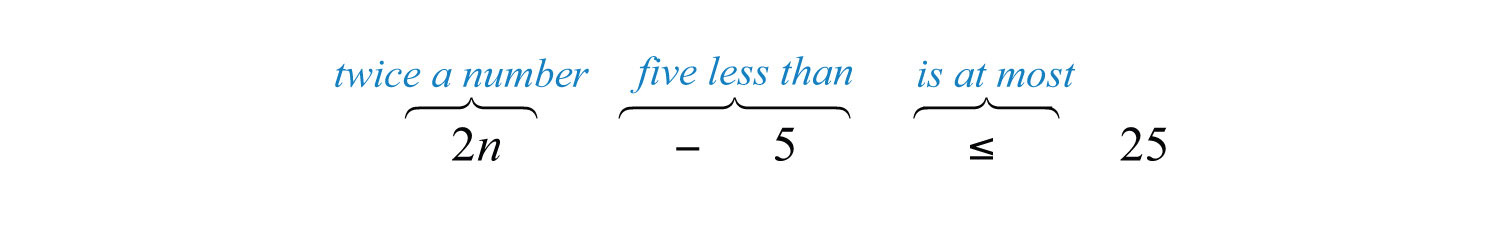Answer: $2n−5≤25$. The key phrase “is at most” indicates that the quantity has a maximum value of 25 or smaller.

Example 10: The temperature in the desert can range from 10°C to 45°C in one 24-hour period. Find the equivalent range in degrees Fahrenheit, F, given that $C=59(F−32)$.

Solution: Set up a compound inequality where the temperature in Celsius is inclusively between 10°C and 45°C. Then substitute the expression equivalent to the Celsius temperature in the inequality and solve for F.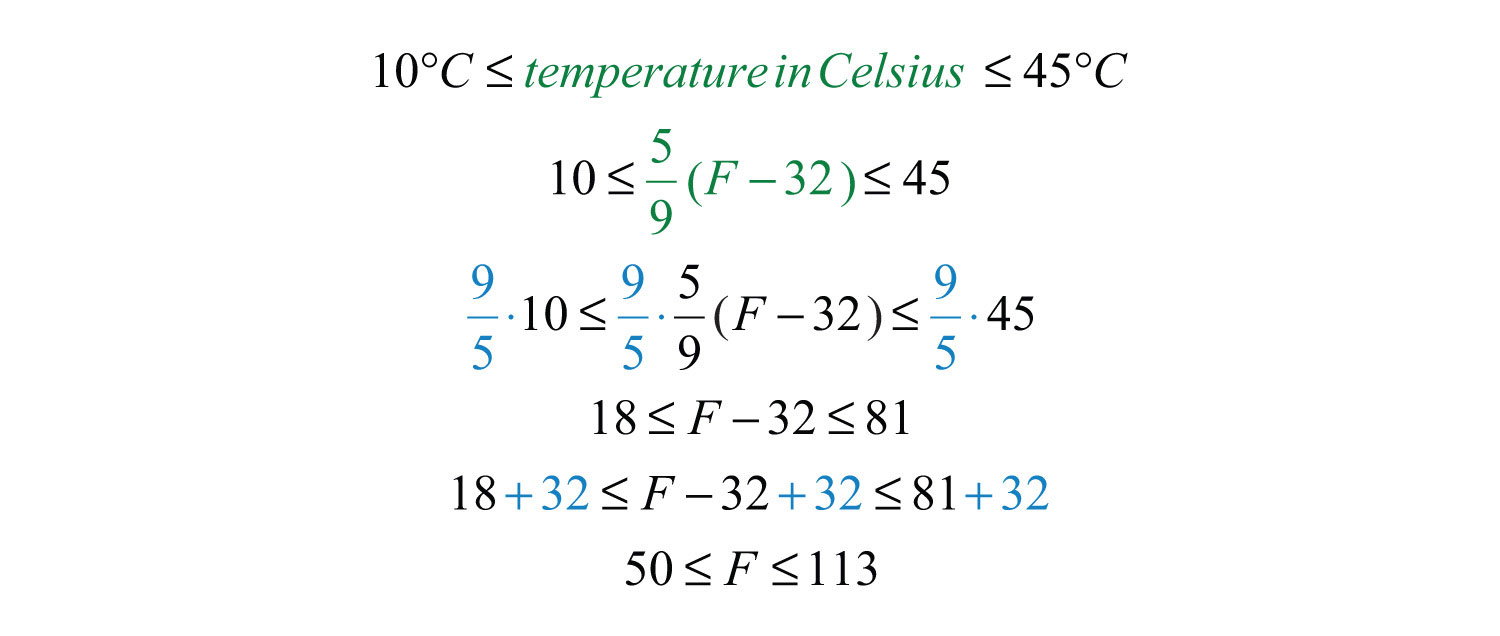Answer: The equivalent Fahrenheit range is from 50°F to 113°F.

Example 11: In the first four events of a meet, a gymnast scores 7.5, 8.2, 8.5, and 9.0. What must she score on the fifth event to average at least 8.5?

Solution: The average must be at least 8.5; this means that the average must be greater than or equal to 8.5.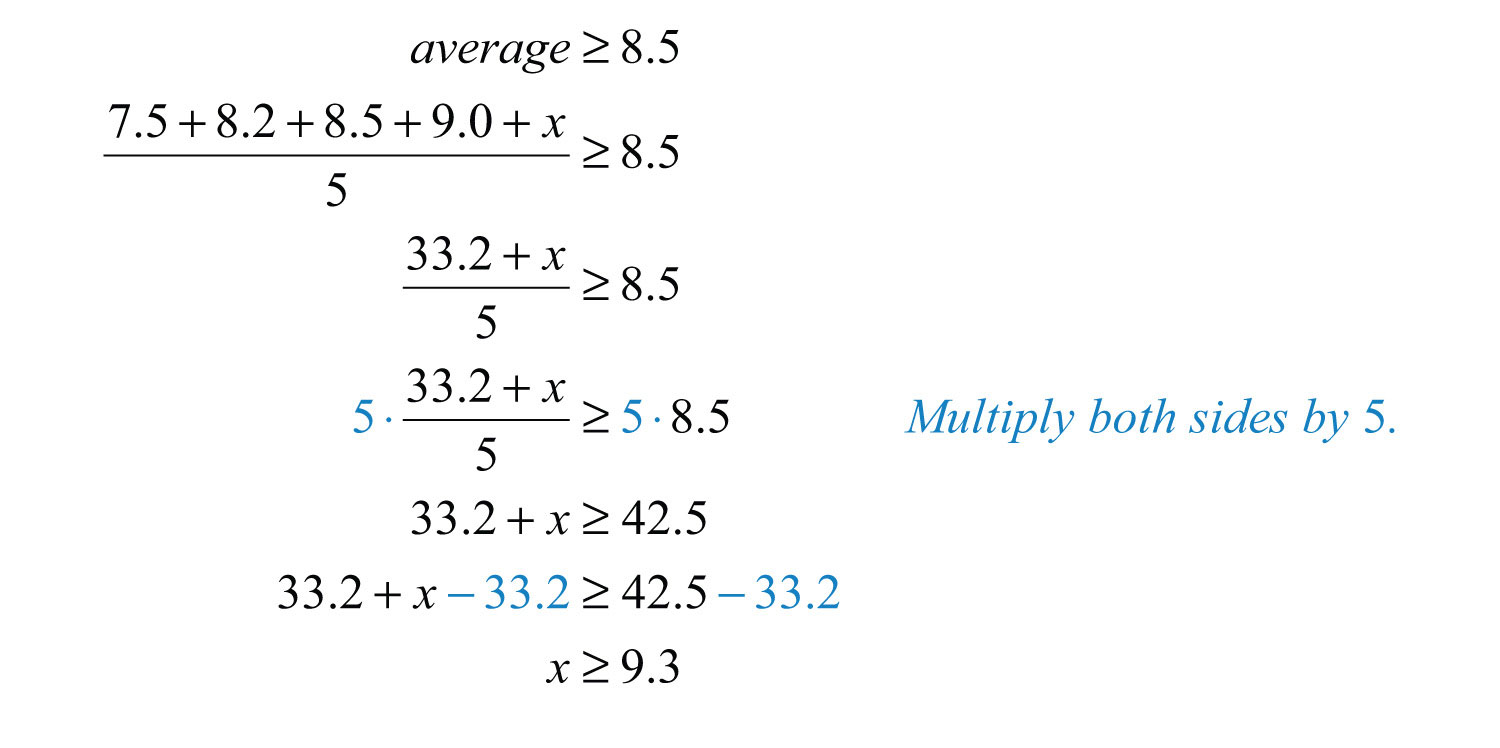Answer: She must score at least 9.3 on the fifth event.

### Key Takeaways

• Inequalities typically have infinitely many solutions. The solutions are presented graphically on a number line or using interval notation or both.
• All but one of the rules for solving linear inequalities are the same as for solving linear equations. If you divide or multiply an inequality by a negative number, reverse the inequality to obtain an equivalent inequality.
• Compound inequalities involving the word “or” require us to solve each inequality and form the union of each solution set. These are the values that solve at least one of the given inequalities.
• Compound inequalities involving the word “and” require the intersection of the solution sets for each inequality. These are the values that solve both or all of the given inequalities.
• The general guidelines for solving word problems apply to applications involving inequalities. Be aware of a new list of key words and phrases that indicate a mathematical setup involving inequalities.

### Topic Exercises

Part A: Checking for Solutions

Determine whether the given number is a solution to the given inequality.

1.

2.

3.

4.

5.

6.

7. ; $x=−10$

8.

9. ; $x=2$

10. ; $x=1$

Part B: Solving Linear Inequalities

Solve and graph the solution set. In addition, present the solution set in interval notation.

11. $x+5>1$

12. $x−3<−4$

13. $6x≤24$

14. $4x>−8$

15. $−7x≤14$

16. $−2x+5>9$

17. $7x−3≤25$

18. $12x+7>−53$

19. $−2x+5<−7$

20. $−2x+4≤4$

21. $−15x+10>20$

22. $−8x+1≤29$

23. $17x−3<1$

24. $12x−13>23$

25. $53x+12≤13$

26. $−34x−12≥52$

27. $−15x+34<−15$

28. $−23x+1<−3$

29. $2(−3x+1)<14$

30. $−7(x−2)+1<15$

31. $9x−3(3x+4)>−12$

32. $12x−4(3x+5)≤−2$

33. $5−3(2x−6)≥−1$

34. $9x−(10x−12)<22$

35. $2(x−7)−3(x+3)≤−3$

36. $5x−3>3x+7$

37. $4(3x−2)≤−2(x+3)+12$

38. $5(x−3)≥15x−(10x+4)$

39. $12x+1>2(6x−3)−5$

40. $3(x−2)+5>2(3x+5)+2$

41. $−4(3x−1)+2x≤2(4x−1)−3$

42. $−2(x−2)+14x<7(2x+1)$

Set up an algebraic inequality and then solve it.

43. The sum of three times a number and 4 is greater than negative 8.

44. The sum of 7 and three times a number is less than or equal to 1.

45. When a number is subtracted from 10, the result is at most 12.

46. When 5 times a number is subtracted from 6, the result is at least 26.

47. If five is added to three times a number, then the result is less than twenty.

48. If three is subtracted from two times a number, then the result is greater than or equal to nine.

49. Bill earns $12.00 for the day plus$0.25 for every person he gets to register to vote. How many people must he register to earn at least $50.00 for the day? 50. With a golf club membership costing$100 per month, each round of golf costs only $25.00. How many rounds of golf can a member play if he wishes to keep his costs to$250 per month at most?

51. Joe earned scores of 72, 85, and 75 on his first three algebra exams. What must he score on the fourth exam to average at least 80?

52. Maurice earned 4, 7, and 9 points out of 10 on the first three quizzes. What must he score on the fourth quiz to average at least 7?

53. A computer is set to shut down if the temperature exceeds 40°C. Give an equivalent statement using degrees Fahrenheit. (Hint: $C=59(F−32)$.)

54. A certain brand of makeup is guaranteed not to run if the temperature is less than 35°C. Give an equivalent statement using degrees Fahrenheit.

Part C: Compound Inequalities

Solve and graph the solution set. In addition, present the solution set in interval notation.

55. $−1

56. $−10≤5x<20$

57. $−2≤4x+6<10$

58. $−10≤3x−1≤−4$

59. $−15<3x−6≤6$

60. $−22<5x+3≤3$

61. $−1≤12x−5≤1$

62. $1<8x+5<5$

63. $−15≤23x−15<45$

64. $−12<34x−23≤12$

65. $−3≤3(x−1)≤3$

66. $−12<6(x−3)≤0$

67. $4<−2(x+3)<6$

68. $−5≤5(−x+1)<15$

69. $−32≤14(12x−1)+34<32$

70. $−4≤−13(3x+12)<4$

71. $−2≤12−2(x−3)≤20$

72. $−5<2(x−1)−3(x+2)<5$

73.

74.

75.

76.

77.

78.

79.

80.

81.

82.

83.

84. $5x+3<4 or 5−10x>4$

85.

86.

87.

88.

Set up a compound inequality for the following and then solve.

89. Five more than two times some number is between 15 and 25.

90. Four subtracted from three times some number is between −4 and 14.

91. Clint wishes to earn a B, which is at least 80 but less than 90. What range must he score on the fourth exam if the first three were 65, 75, and 90?

92. A certain antifreeze is effective for a temperature range of −35°C to 120°C. Find the equivalent range in degrees Fahrenheit.

93. The average temperature in London ranges from 23°C in the summer to 14°C in the winter. Find the equivalent range in degrees Fahrenheit.

94. If the base of a triangle measures 5 inches, then in what range must the height be for the area to be between 10 square inches and 20 square inches?

95. A rectangle has a length of 7 inches. Find all possible widths if the area is to be at least 14 square inches and at most 28 square inches.

96. A rectangle has a width of 3 centimeters. Find all possible lengths, if the perimeter must be at least 12 centimeters and at most 26 centimeters.

97. The perimeter of a square must be between 40 feet and 200 feet. Find the length of all possible sides that satisfy this condition.

98. If two times an angle is between 180 degrees and 270 degrees, then what are the bounds of the original angle?

99. If three times an angle is between 270 degrees and 360 degrees then what are the bounds of the original angle?

Part D: Discussion Board Topics

100. Research and discuss the use of set-builder notation with intersections and unions.

101. Can we combine logical “or” into one statement like we do for logical “and”?

1: Yes

3: No

5: Yes

7: Yes

9: Yes

11: $x>−4$; $(−4, ∞)$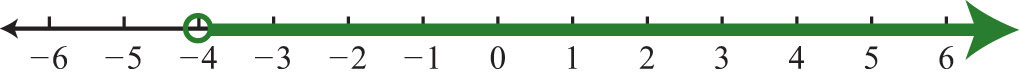13: $x≤4$; $(−∞, 4]$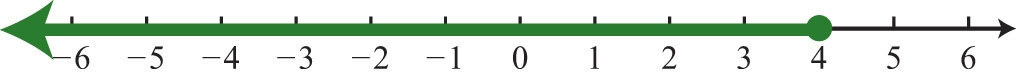15: $x≥−2$; $[−2, ∞)$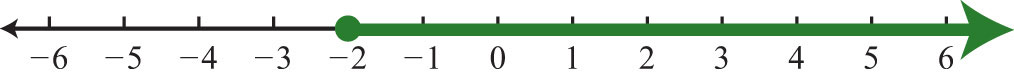17: $x≤4$; $(−∞, 4]$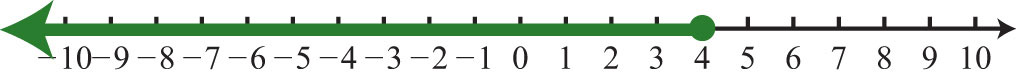19: $x>6$; $(6, ∞)$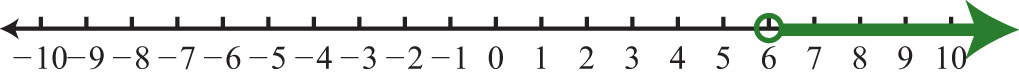21: $x<−23$; $(−∞, −23)$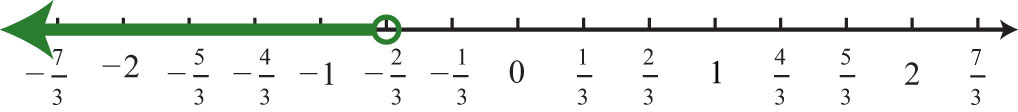23: $x<28$; $(−∞, 28)$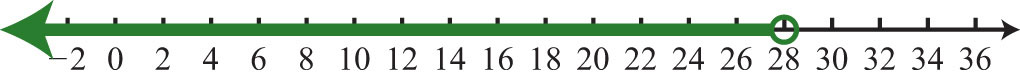25: $x≤−110$; $(−∞, −110]$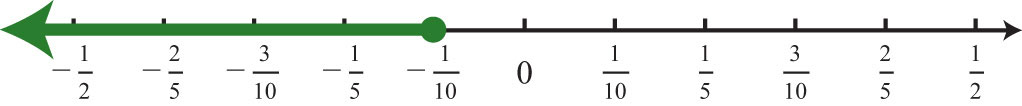27: $x>194$; $(194, ∞)$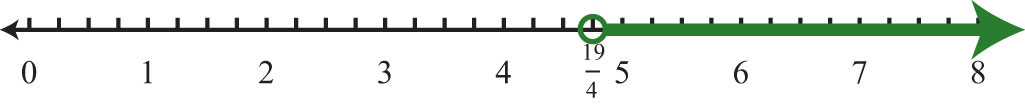29: $x>−2$; $(−2, ∞)$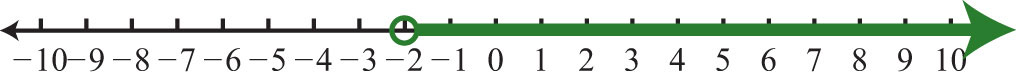31: $∅$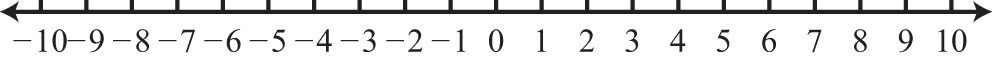33: $x≤4$; $(−∞, 4]$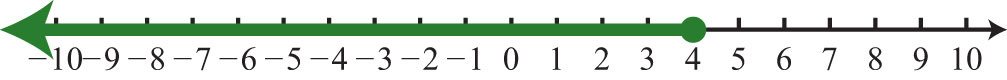35: $x≥−20$; $[−20, ∞)$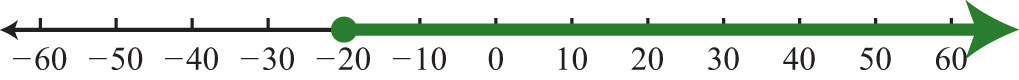37: $x≤1$; $(−∞, 1]$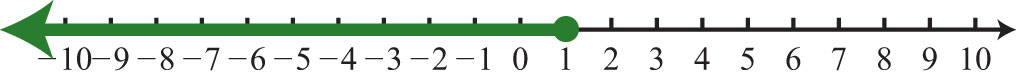39: R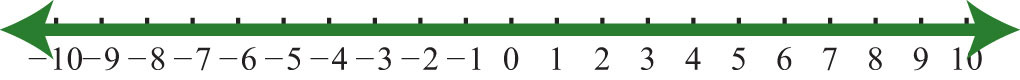41: $x≥12$; $[12, ∞)$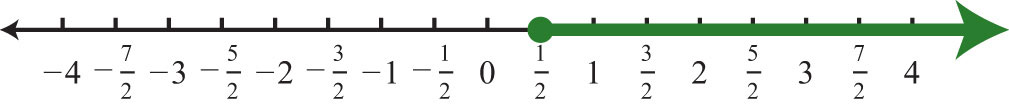43: $n>−4$

45: $n≥−2$

47: $n<5$

49: Bill must register at least 152 people.

51: Joe must earn at least an 88 on the fourth exam.

53: The computer will shut down when the temperature exceeds 104°F.

55: $−4; $(−4, 2)$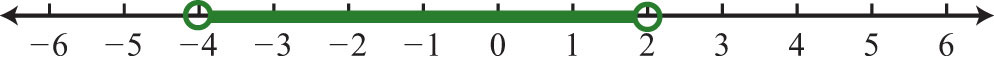57: $−2≤x<1$; $[−2, 1)$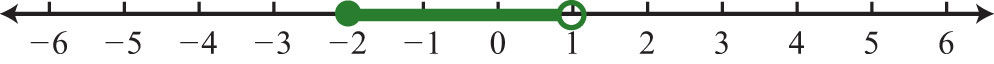59: $−3; $(−3, 4]$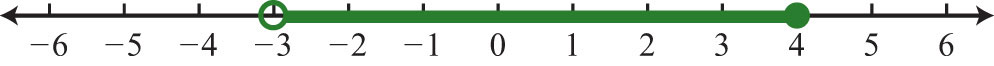61: $8≤x≤12$; $[8, 12]$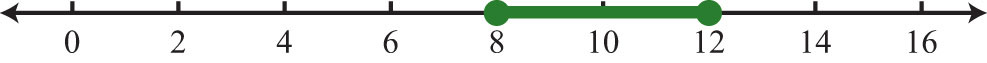63: $0≤x<32$; $[0, 32)$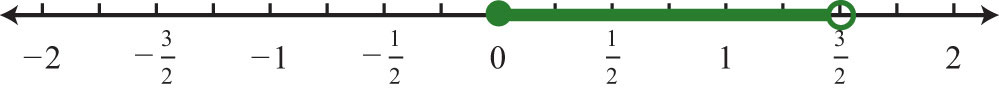65: $0≤x≤2$; $[0, 2]$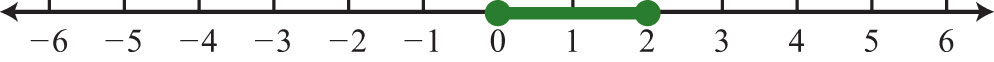67: $−6; $(−6, −5)$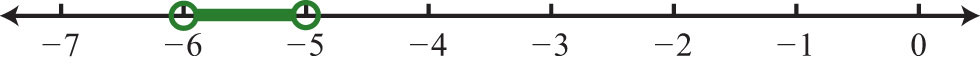69: $−16≤x<8$; $[−16, 8)$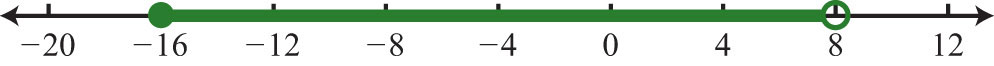71: $−1≤x≤10$; $[−1, 10]$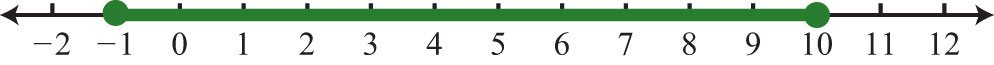73: ; $(−∞, −5]∪(3, ∞)$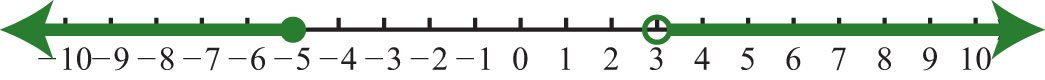75: ; $(−∞, 0)∪(1, ∞)$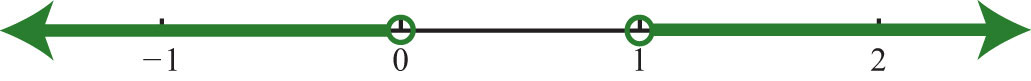77: R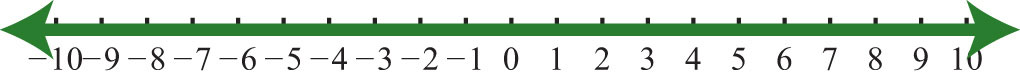79: ; $(−∞, 2)∪(6, ∞)$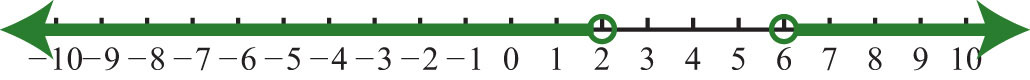81: $x≤0$; $(−∞, 0]$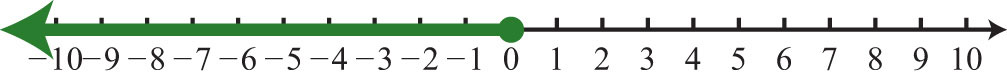83: $x<4$; $(−∞, 4)$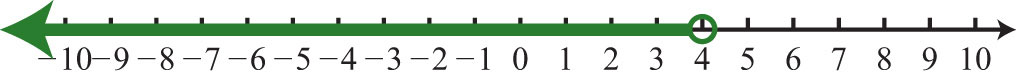85: $−4; $(−4, 6)$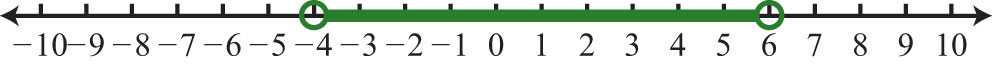87: $x<3$; $(−∞, 3)$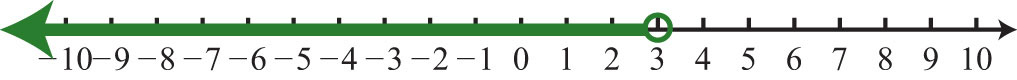89: $5

91: Clint must earn a score in the range from 90 to 100.

93: The average temperature in London ranges from 57.2°F to 73.4°F.

95: The width must be at least 2 inches and at most 4 inches.

97: Sides must be between 10 feet and 50 feet.

99: The angle is between 90 degrees and 120 degrees.